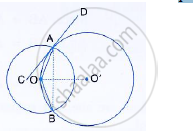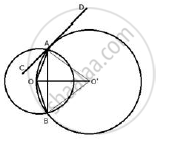Share

# Two Circle with Centres O and O ' Are Drawn to Intersect Each Other at Points a and B.Prove that Oa Bisects Angle Bac. - Mathematics

Course

#### Question

Two circle with centres O and O ' are drawn to intersect each other at points A and B.
Centre O of one circle lies on the circumference of the other circle and CD is drawn tangent to the circle with centre O ' at A. prove that OA bisects angle BAC.#### SolutionJoin OA, OB, O'A, O'B and O'O.
CD is the tangent and AO is the chord.
∠OAC = ∠OBA (angles in alternate segment)
In ΔOAB ,
OA = OB (Radii of the same circle)
∴ OAB = ∠OBA …..(ii)
From (i) and (ii)
∠OAC = ∠OAB
Therefore, OA is bisector of ∠BAC

Is there an error in this question or solution?